# Winter’s Method HelpThe Winter’s Method time series analysis is used to analyze data that has a trend and a seasonal component. There are two models for The Winter’s Method: multiplicative and additive. Both models are shown below.

Winter’s Method (Multiplicative)

Lt = α(Yt/St-p) + (1 – α)(Lt-1 + Tt-1)

Tt = g(Lt – Lt-1) + (1 – g)Tt-1

St = d(Yt/Lt) + (1 – d)St-p

Ŷt= (Lt-1 + Tt-1)St-p

Lt =α(Yt – St–p) + (1 – α)(Lt–1 + Tt–1)

Tt =g(Lt – Lt–1) + (1 – g)Tt–1

St =d(Yt – Lt) + (1 – d)St–p

Ŷt= Lt–1 + Tt–1 + St–p

where:

• Lt = Level component at time t
• α= level weight
• Tt = Trend component at time t
• g= trend weight
• St = Seasonal component at time t
• d= seasonal weight
• p = seasonal period
• Ŷt= fitted value at time t

This help page describes how to perform the Winter’s Method time series analysis using the SPC for Excel software with the data that can be downloaded at this link. This page contains the following:

### Data Entry

Enter your data in a spreadsheet as shown below. The data must be in a single column with or without a heading. The data can be anywhere in the spreadsheet. There can be no empty cells or non-numeric entries in the data (except if there is a heading). It is important that the data represent evenly spaced data in time.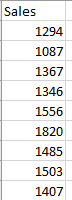### Running the Analysis

Select the data on the worksheet to be included in the analysis. You just have to put the cursor in the first row (the data or the heading as shown above). The software will select the data automatically then.

To start the analysis, select “Time Series” under the “Analysis” panel on the SPC for Excel ribbon. The form below is shown.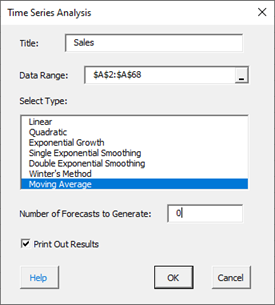• Title: this is the data name; the default is the first cell in the data column if it is non-numeric; otherwise it is blank.
• Data Range: the range containing the data; if this range is not correct, it can be edited here.
• Select Type: select the time series method you wish to use; Winter’s Method in this example
• Number of Forecasts to Generate: enter the number of forecasts you want; the default is 0.
• Print out Results: this option prints out the results in a spreadsheet including the model, MAPE, MAD, MSD, the actual values, the fitted values, the residuals, and the forecasts if any; the default is to print out the results. This output is in addition to the chart that is created (see below).
• Select Cancel to stop the analysis.
• Select OK to create to show the next form below.• Seasonal Length: enter the season length, 4 for quarters or 12 for years for example.
• Select Model:
• Multiplicative: select this option to use the multiplicative model; this is the default.
• Weight Options:
• Optimize Level, Trend and Seasonal Weights: this option allows the software to find the values of the level weight (), trend weight () and seasonal weight () in the above equations that minimize the sum of the errors squared.
• Enter Level, Trend and Seasonal Weights: this options allows you to enter the value you want for the Level, Trend, and Seasonal weights.
• Select Cancel to end.
• Select OK to create the output.

### Output

The output from the Winter’s Method time series analysis consists of two parts: the chart and the printed results (if that option was selected).

The Winter’s Method chart is shown below. It includes the actual values, the fitted values, the forecasts (if a number greater than 0 was entered; 6 was used in this example), the values of MAPE, MAD, and MSD and the weights.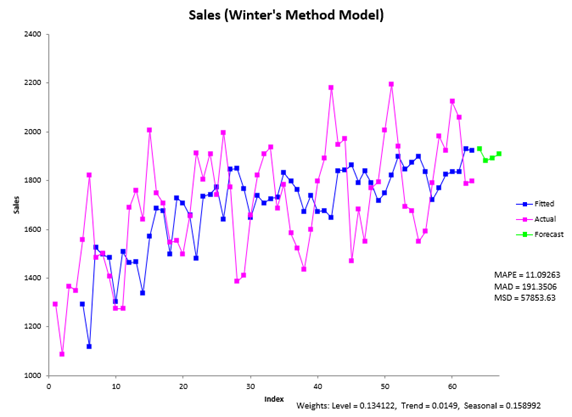The forecast values for the next k periods are given by:

Multiplicative method: (Lt + kTt) * St + k −p

Additive method: Lt + kTt +St + m −p

The 95% prediction limits for the multiplicative method are given by:1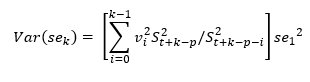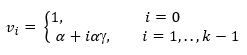Upper Prediction Limit = Forecast + 1.96 × [Var(et(k))]1/2
Lower Prediction Limit = Forecast – 1.96 ×[Var(et(k))]1/2

wherek = number of steps ahead andσe2= Var(et(l)) = the variance of the one step ahead forecast errors, which is estimated from the data used for fitting.

The 95% prediction limits for the additive method are given by:2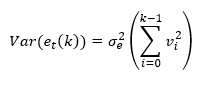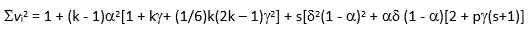Upper Prediction Limit = Forecast + 1.96 × [Var(et(k))]1/2
Lower Prediction Limit = Forecast – 1.96 ×[Var(et(k))]1/2

wherek = number of steps ahead andσe2= Var(et(l)) = the variance of the one step ahead forecast errors, which is estimated from the data used for fitting.

The printed results are shown below. It includes the same information as in the chart but in tabular format.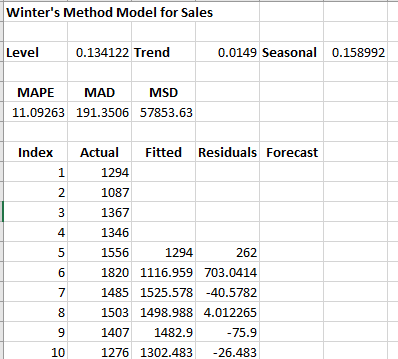### Interpretation of Output

The first thing to do is to examine the chart of the fitted values with the actual values. Decide if the fitted values fit the data. If so, then it is probably a good fit. If you ran other models, you compare the three accuracy measures (MAPE, MAD, and MSD) described in the first time series help page. The smaller the values, the better the fit.

If there are forecasts values, you can check to see how good they might be. You want the fitted line to closely follow the actual results. If it begins to vary at the end of the actual data, something may be changing (like the trend or the seasonal components).

1Prediction Intervals for Multiplicative Holt-Winters, Chatfied and Yar, International Journal of Forecasting 7 (1991)

2Prediction Intervals fro the Holt-Winters Forecasting Procedure; Chatfield and Yar, International Journal of Forecasting 6 (1990)